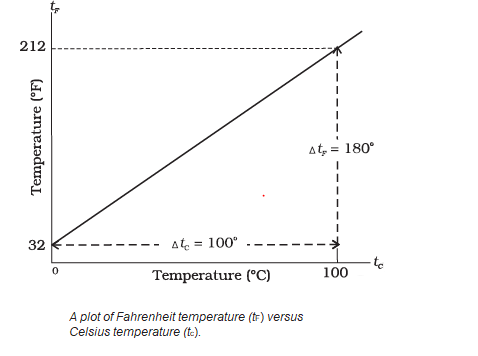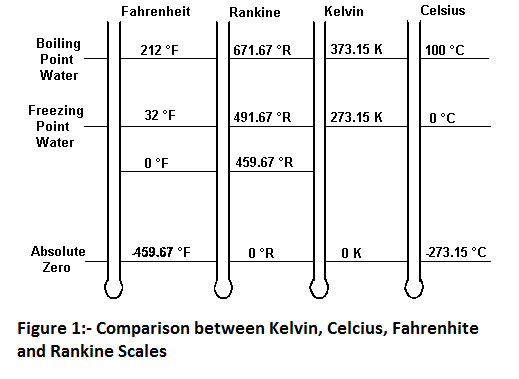# Measurement of Temperature

## Measurement of temperature

Measurement of temperature of a body is very important. It is also necessary to be able to measure both high and low temperatures. To make this possible it is necessary to construct a suitable scale of temperature. The scale chosen must be precise and consistent. The temperatures measured on this scale must be accurate. Measurement of Temperature can be obtained using a thermometer. A thermometer, such as mercury in glass thermometer, is a device whose readings depends on hotness or coldness of an object. A thermometer is a reliable device for measurement of temperature than our senses.
The temperature of an object is not a fixed number but depends on the type of thermometer and on the temperature scale adopted. Construction of thermometers generally requires a measurable property of a substance which monotonically changes with temperature. Examples of some common type of thermometers:
1. Mercury in a glass thermometer:- The height of mercury in the tube is taken as thermometric parameter.
2. Constant Volume gas thermometer:- Gas in bulb is maintained at constant volume. The mean pressure of gas is taken as thermometric parameter.
3. Constant Pressure gas thermometer:- Gas in bulb is maintained at constant pressure. Volume of gas is taken as thermometric parameter.
4. Resistance thermometer:- Electric resistance of a metal wire increases monotonically with the temperature and may be used to define temperature scale. Such thermometers are resistance thermometers. Electric resistance of metal wire increase monotonically with temperature and may be used to define the temperature scale. If $R_{0}$ and $R_{100}$ are resistance of metal wire at ice and steam point respectively then temperature t can be defined corresponding to resistance $R_{T}$ as follows $$T=\frac{(R_{T}-R_{0})100}{(R_{100}-R_{0})}$$ A platinum wire is often used to construct a thermometer which is known as platinum resistance thermometer.
Some other type of thermometers are radiation thermometers, bimetallic thermometers , magnetic thermometers etc. Thermometers are calibrated to assign a numerical value to any given temperature. Definition of any standard scale needs two fixed reference points and these points can be correlated to physical phenomenon reproducible at the same temperature. Two such standard points are freezing and boiling points of water at same pressure. Two such familiar scales used for measurement of temperature are Celsius and Fahrenheit scale. Temperature in Celsius is measured in degree. Fahrenheit scale has a smaller degree then Celsius scale and a different zero of temperature. Relation between Celsius and Fahrenheit scale is
$$T_{F}=\frac{9}{5}T_{C}+32^{\circ}$$ Where, $T_{F}$ temperature in Fahrenheit and $T_{C}$ – Temperature in Celsius.
Letters $C$ and $F$ are used to distinguish measurements on two scales thus, $0^{\circ}C=32^{\circ} F$ this means that $0^{\circ}$ C on Celsius scale measures the same temperature as $32^{\circ}$ F on the Fahrenheit scale. The plot between Fahrenheit temperature ($t_F$) versus Celsius temperature($t_C$)On Fahrenheit scale melting point of ice and boiling point of water have values $32^{\circ}F$ and $212^{\circ}F$ and that on Celsius scale are $0^{\circ} C$ and $100^{\circ} C$.
If we now talk of Kelvin scale ,the melting point of ice and boiling point of water in the scale are 273.15 K and 373.15 K respectively.Size of a degree in Celsius and kelvin scale are same. Relation between Celsius and kelvin scale is
$$T_{C} = T_{K} - 273.15 K$$ where, $T_{K}$ is Temperature in Kelvin and $T_{C}$ - Temperature in Celsius.Above figure shows the temperatures of the absolute zero, meting point of ice and the boiling point of water as measured on the Celsius, Kelvin , Fahrenheit and Rankine scales. Celsius and Fahrenheit scales show the same reading at $-40{^0}$ that is $-40{^0}C=-40{^0}F$. The Kelvin scale and Rankine scale agree at zero degree only.
Relation
$$\frac{C-0}{100}=\frac{F-32}{180}=\frac{K-273}{100}=\frac{R-492}{180}$$ Also, $$\frac{K}{100}=\frac{R}{180}$$

### Solved Examples

Question 1 The temperature of the surface of sun is about $6500^{0}C$. What is the temperature on (i) the Rankine scale and (ii) on the Kelvin Scale?
Solution Here $C=6500^{0}C$
We have the relation ,
\begin{align*} & \frac{C-0}{100}=\frac{K-273}{100}=\frac{R-492}{180} \end{align*} Putting the value of $C$ in above relation We get \begin{align*} & \frac{6500}{100}=\frac{K-273}{100}=\frac{R-492}{180} \\ & K=6500+273=6773 K \\ & R=12,192^{0}R \end{align*} The temperature of the surface of sun corresponding to $C=6500^{0}C$ is (i) $6773 K$ and (ii)$R=12,192^{0}R$